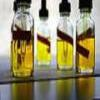You may also likeMathematical Issues for Chemists

A brief outline of the mathematical issues faced by chemistry students.Reaction Rates

Explore the possibilities for reaction rates versus concentrations with this non-linear differential equationCatalyse That!

Can you work out how to produce the right amount of chemical in a temperature-dependent reaction?

Mixing pH

Why do this problem?

This problem provides a good exercise in linking two areas of mathematics: concentration and logarithms. Students will need to flip between the two descriptions of acidity which will require careful, clear thinking. The context is fascinating and this will encourage a 'concrete' and practical engagement with logarithms.

Possible approach

This question uses the advanced concept of logarithms but will also use basic ideas about concentrations and mixtures. Students will need to remember basic ideas about the meaning of a 'concentration' in order to work out the concentration of mixtures.

Key questions

What equations do you need to flip between the pH and the concentration of $H^+$ ions?
How can you work out the concentration of a mixture of two solutions?

Possible extension

Try the next question in the sequence: Extreme dissociation

Possible support

You might try and get started using the pH formula with the problem Temperature pH